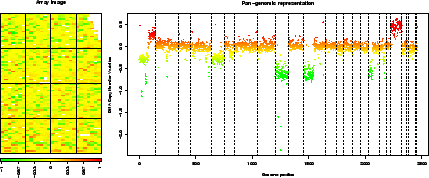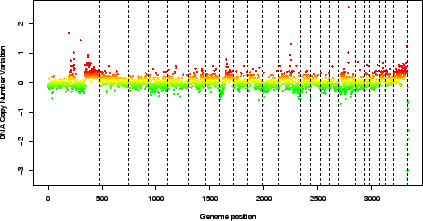Subsections

# 7 Sample MANOR sessions

In this section we illustrate the use of MANOR on two CGH arrays. Our examples contain several steps, including data preparation, flag definition, array normalization, quality criteria definition, and quality assessment of the array, and highlights of the normalization process.

## 7.1 array edge

### 7.1.1 Data preparation: import

```> dir.in <- system.file("data", package = "MANOR")
> spot.names <- c("LogRatio", "RefFore", "RefBack", "DapiFore",
+     "DapiBack", "SpotFlag", "ScaledLogRatio")
> clone.names <- c("PosOrder", "Chromosome")
> edge <- import(paste(dir.in, "/edge.txt", sep = ""), type = "spot",
+     spot.names = spot.names, clone.names = clone.names, add.lines = TRUE)
 "number of lines does not match array design: adding empty lines..."
```

### 7.1.2 Normalization: norm.arrayCGH

Figure 6 shows the results of the normalization process.
```> data(flags)
> data(spatial)
> local.spatial.flag\$args <- alist(var = "ScaledLogRatio", by.var = NULL,
+     nk = 5, prop = 0.25, thr = 0.15, beta = 1, family = "gaussian")
> flag.list <- list(spatial = local.spatial.flag, spot = spot.corr.flag,
+     ref.snr = ref.snr.flag, dapi.snr = dapi.snr.flag, rep = rep.flag,
+     unique = unique.flag)
> edge.norm <- norm.arrayCGH(edge, flag.list = flag.list, FUN = median,
+     na.rm = TRUE)
 "spatial"
 "mean of unbiased zone :  -0.0231566395663957"
 "Spatial bias has been detected"
zone.number           mu effectif effectif.cumul frequency.cumul biased.zone
4           5  0.467833333       66             66      0.00918964           1
3           4  0.045546490     1581           1647      0.22932331           0
5           3  0.004946157     2693           4340      0.60428850           0
1           2 -0.034216274     1868           6208      0.86438318           0
2           1 -0.079646817      974           7182      1.00000000           0
 "spot"
 "ref.snr"
 "dapi.snr"
 "rep"
 "unique"
> edge.norm <- sort.arrayCGH(edge.norm, position.var = "PosOrder")
```

 ```> report.plot(edge.norm, chrLim = "LimitChr", zlim = c(-1, 1)) ```### 7.1.3 Quality assessment: qscore.summary.arrayCGH

```> profileCGH <- as.profileCGH(edge.norm\$cloneValues)
+     model = "Gaussian", qlambda = 0.999, bandwidth = 10, base = FALSE,
+     round = 2, lambdabreak = 6, lambdaclusterGen = 20, param = c(d = 6),
+     alpha = 0.001, msize = 5, method = "centroid", nmin = 1,
+     nmax = 8, amplicon = 1, deletion = -5, deltaN = 0.1, forceGL = c(-0.15,
+         0.15), nbsigma = 3, MinBkpWeight = 0.35, verbose = FALSE)
 "Smoothing for each Chromosome"
 "Optimization of the Breakpoints"
 "Check Breakpoints Position"
> edge.norm\$cloneValues <- as.data.frame(profileCGH)
> edge.norm\$cloneValues\$ZoneGNL <- as.factor(edge.norm\$cloneValues\$ZoneGNL)
> data(qscores)
> qscore.list <- list(smoothness = smoothness.qscore, var.replicate = var.replicate.qscore,
+     dynamics = dynamics.qscore)
> edge.norm\$quality <- qscore.summary.arrayCGH(edge.norm, qscore.list)
> edge.norm\$quality
name                                     label score
1 LOCAL_SMOOTHNESS Local signal variability along the genome 0.021
2    VAR_REPLICATE      Average variability among replicates 0.011
3  SIGNAL_DYNAMICS Dynamics of the DNA copy number variation 0.399
```

### 7.1.4 Highlights of the normalization process: html.report

Function html.report generates an HTML file with key features of the normalization process: array image and genomic profile before and after normalization, spot-level flag report, and value of the quality criteria.

```> html.report(edge.norm, dir.out = ".", array.name = "an array with local bias",
+     chrLim = "LimitChr", light = FALSE, pch = 20, zlim = c(-2,
+         2), file.name = "edge")
```

The results of the previous command can be viewed in the file edge.html.

Here we give the example of the normalization of an array with spatial gradient.

### 7.2.1 Data preparation: import

```> spot.names <- c("Clone", "FLAG", "TEST_B_MEAN", "REF_B_MEAN",
+     "TEST_F_MEAN", "REF_F_MEAN", "ChromosomeArm")
> clone.names <- c("Clone", "Chromosome", "Position", "Validation")
> ac <- import(paste(dir.in, "/gradient.gpr", sep = ""), type = "gpr",
+     spot.names = spot.names, clone.names = clone.names, sep = "\t",
+     comment.char = "@", add.lines = TRUE)
 "number of lines does not match array design: adding empty lines..."
 "calculating array design..."
> ac\$arrayValues\$F1 <- log(ac\$arrayValues[["TEST_F_MEAN"]], 2)
> ac\$arrayValues\$F2 <- log(ac\$arrayValues[["REF_F_MEAN"]], 2)
> ac\$arrayValues\$B1 <- log(ac\$arrayValues[["TEST_B_MEAN"]], 2)
> ac\$arrayValues\$B2 <- log(ac\$arrayValues[["REF_B_MEAN"]], 2)
> Ratio <- (ac\$arrayValues[["TEST_F_MEAN"]] - ac\$arrayValues[["TEST_B_MEAN"]])/(ac\$arrayValues[["REF_F_MEAN"]] -
+     ac\$arrayValues[["REF_B_MEAN"]])
> Ratio[(Ratio <= 0) | (abs(Ratio) == Inf)] <- NA
> ac\$arrayValues\$LogRatio <- log(Ratio, 2)
```

### 7.2.2 Normalization: norm.arrayCGH

Figure 7 shows the results of the normalization process.

```> data(spatial)
> data(flags)
> flag.list <- list(local.spatial = local.spatial.flag, spot = spot.flag,
+     SNR = SNR.flag, global.spatial = global.spatial.flag, val.mark = val.mark.flag,
+     position = position.flag, unique = unique.flag, amplicon = amplicon.flag,
+     chromosome = chromosome.flag, replicate = replicate.flag)
+     FUN = median, na.rm = TRUE)
 "local.spatial"
 "mean of unbiased zone :  8.39530436452067"
 "There is no spatial bias"
zone.number       mu effectif effectif.cumul frequency.cumul biased.zone
1           7 8.693635      510            510      0.05083732           0
2           6 8.606773      626           1136      0.11323764           0
3           5 8.494905     1083           2219      0.22119219           0
4           4 8.469920     2510           4729      0.47139155           0
5           3 8.409388     2520           7249      0.72258772           0
6           2 8.349206     2222           9471      0.94407895           0
7           1 8.180788      561          10032      1.00000000           0
 "spot"
 "SNR"
 "global.spatial"
 "val.mark"
 "position"
 "unique"
 "amplicon"
 "chromosome"
 "replicate"
```

 ```> genome.plot(gradient.norm, chrLim = "LimitChr") ```### 7.2.3 Quality assessment: qscore.summary.arrayCGH

```> profileCGH <- as.profileCGH(gradient.norm\$cloneValues)
+     model = "Gaussian", qlambda = 0.999, bandwidth = 10, base = FALSE,
+     round = 2, lambdabreak = 6, lambdaclusterGen = 20, param = c(d = 6),
+     alpha = 0.001, msize = 5, method = "centroid", nmin = 1,
+     nmax = 8, amplicon = 1, deletion = -5, deltaN = 0.1, forceGL = c(-0.15,
+         0.15), nbsigma = 3, MinBkpWeight = 0.35, verbose = FALSE)
 "Smoothing for each Chromosome"
 "Optimization of the Breakpoints"
 "Check Breakpoints Position"
> data(qscores)
> qscore.list <- list(smoothness = smoothness.qscore, var.replicate = var.replicate.qscore,
+     dynamics = dynamics.qscore)
+     qscore.list)
name                                     label score
1 LOCAL_SMOOTHNESS Local signal variability along the genome 0.032
2    VAR_REPLICATE      Average variability among replicates 0.050
3  SIGNAL_DYNAMICS Dynamics of the DNA copy number variation 0.294
```

### 7.2.4 Highlights of the normalization process: html.report

Function html.report generates an HTML file with key features of the normalization process: array image and genomic profile before and after normalization, spot-level flag report, and value of the quality criteria.

```> html.report(gradient.norm, dir.out = ".", array.name = "an array with spatial gradient",
+     chrLim = "LimitChr", light = FALSE, pch = 20, zlim = c(-2,